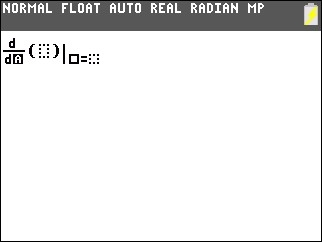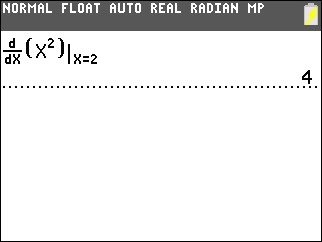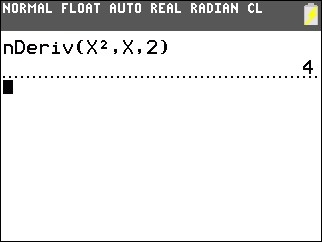# Knowledge Base

## Solution 34918: Calculating Derivatives on the TI-84 Plus Family of Graphing Calculators.

### How can I calculate derivatives on the TI-84 Plus family of graphing calculators?

The TI-84 Plus family of graphing calculators can only calculate numeric derivatives. Please refer to the example below.

Example: Find the numeric derivative of f(x)=x² at x=2

Using MATHPRINT Mode:

1) Press [MATH].
2) Press [↓] until 8:nDeriv( is selected and press [ENTER].3) Press [X,T,θ,n] [X,T,θ,n] [x²] [→]  [ENTER].Using CLASSIC mode:

1) Press [MATH].
2) Press [↓] until 8:nDeriv( is selected and press [ENTER].
3) Press [X,T,θ,n] [x²] [,] [X,T,θ,n] [,]  [)] [ENTER].Please Note: The TI-84 Plus family of graphing calculators do not have symbolic manipulation capabilities and cannot find the symbolic derivative of a function. For a list of TI graphing calculators that have the Computer Algebraic System (CAS) please refer to KB#24631.